# Period of Oscillation Equation Sample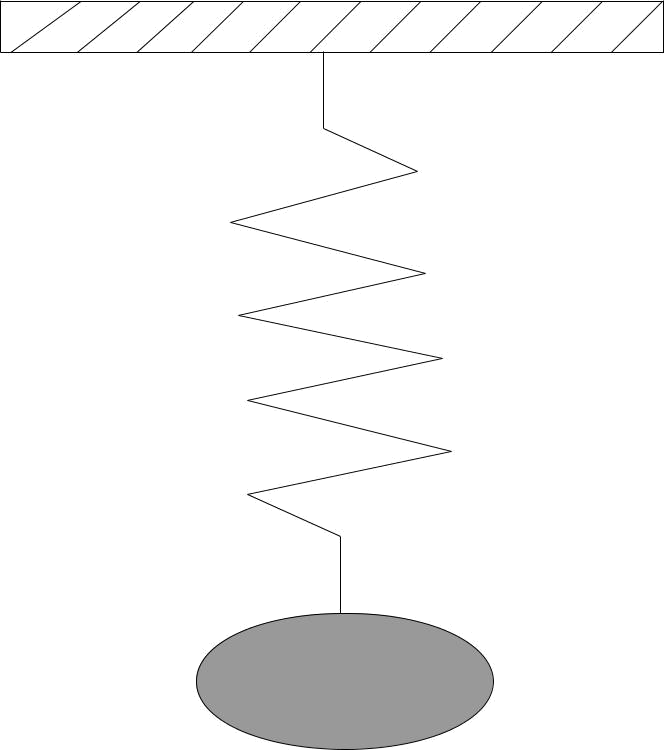A block hanging down from a spring has a mass of 5 kg. The spring stiffness constant is 1000 N/m.

The block was displaced from the equilibrium state by 10 cm and released.

Find the amplitude, period, and angular frequency.

Determine the velocity and acceleration of the block at 5 cm distance from the equilibrium position.

Solution:

The amplitude of the block is the maximum distance that the block travels from its equilibrium state:

A=10 cm=0.1 m

The angular frequency can be found from the following equation: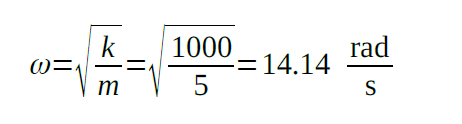To find the period we need to find the frequency first: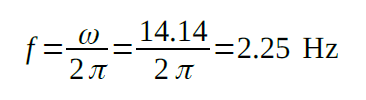Now the period can be determined: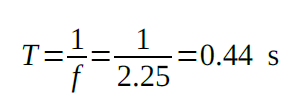It is known that the velocity is the first order derivative of the displacement function: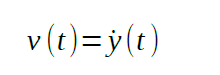The acceleration is the first order derivative of the velocity function: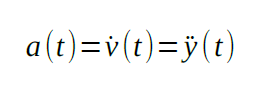The displacement function can be written as: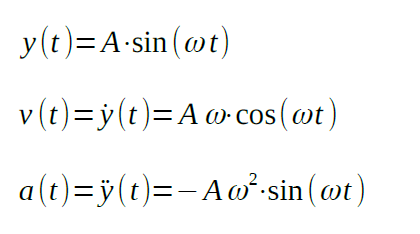Knowing the distance, we can find the time at which the block will be at that point: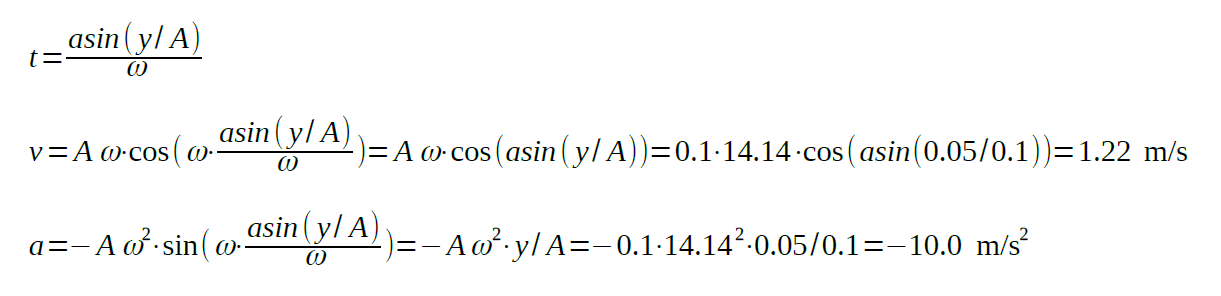If you study physics, then this sample period of oscillation equation will help you. We hope that it is understandable and clear to you. There are different types of samples that you can find on our blog. You will perform better if you use them, as they contain useful ideas.

If you need to deal with an amplitude of oscillation equation, get help from one of our experts. If homework causes huge amounts of stress for you, then you need our professional help. We have experts who are knowledgeable in different spheres of study. Get the solution for your problem right here!